# Calculus 1 : How to find trapezoidal approximation by graphing functions

## Example Questions

← Previous 1

### Example Question #1 : How To Find Trapezoidal Approximation By Graphing Functions

Use the trapezoidal approximation to find the area under the curve using the graph with four partitions.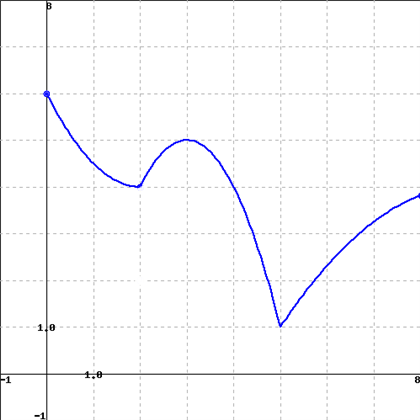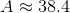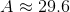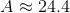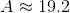Explanation:

The trapezoid rule states that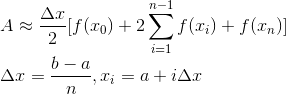.

Therefore, using our graph, we have: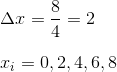We find the function values at the sample point: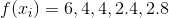Then we substitute the appropriate values into the trapezoid rule approximation: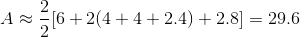### Example Question #2 : Trapezoidal Approximation

Is the following function increasing or decreasing at the point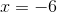?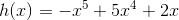h(x) is increasing at, because the first derivative is positive.

h(x) is increasing at, because the second derivative is positive.

h(x) is decreasing at, because the first derivative is negative.

h(x) is decreasing at, because the second derivative is negative.

h(x) is decreasing at, because the first derivative is negative.

Explanation:

Is the following function increasing or decreasing at the point?Increasing and decreasing intervals can be found via the first derivative. Since derivatives measure rates of change, the sign of the derivative at a given point can tell you whether a function is increasing or decreasing.

Begin by taking the derivative of our function:Becomes: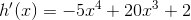Next, find h'(-6) and look at the sign.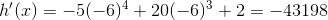So, our first derivative is very negative at the given point. This means that h(x) is decreasing.

### Example Question #3 : Trapezoidal Approximation

Use the trapezoidal approximation to approximate the following integral: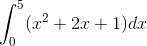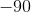Explanation:

The trapezoidal approximation of a definite integral is given by the following formula: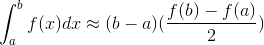Using the above formula, we get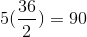### Example Question #4 : Trapezoidal Approximation

Use the trapezoidal approximation to evaluate the following integral: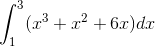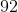Explanation:

To evaluate a definite integral using the trapezoidal approximation, we must use the formulaUsing the above formula, we get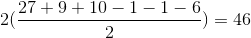### Example Question #5 : Trapezoidal Approximation

Use the trapezoidal approximation to evaluate the following integral: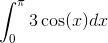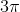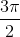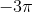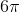Explanation:

To evaluate a definite integral using the trapezoidal approximation, we must use the formula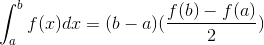Using the above formula, we get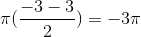### Example Question #6 : Trapezoidal Approximation

Evaluate the following integral using the trapezoidal approximation: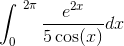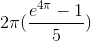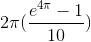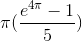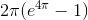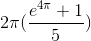Explanation:

To evaluate the integral using the trapezoidal rule, we must use the formulaUsing the above formula, we get the following:### Example Question #7 : Trapezoidal Approximation

Evaluate the integral using the trapezoidal approximation: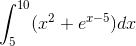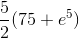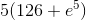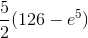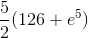Explanation:

To evaluate a definite integral using the trapezoidal approximation, we must use the following formula: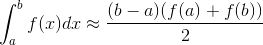So, using the above formula, we get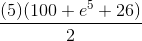which simplifies to### Example Question #8 : Trapezoidal Approximation

Evaluate the integral using the trapezoidal approximation: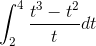Explanation:

To evaluate the definite integral using the trapezoidal approximation, we must use the following formula: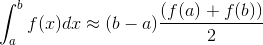Using the above formula, we get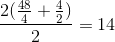### Example Question #9 : Trapezoidal Approximation

Evaluate the integral using the trapezoidal approximation: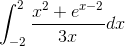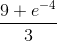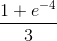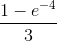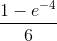Explanation:

To evaluate the integral using the trapezoidal approximation, we must use the following formula: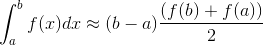Using the formula, we get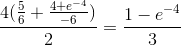### Example Question #10 : Trapezoidal Approximation

Evaluate the integral using the trapezoidal approximation: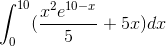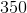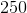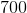Explanation:

To evaluate the definite integral using the trapezoidal approximation, the following formula is used:Using the above formula, we get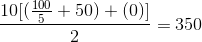.

← Previous 1